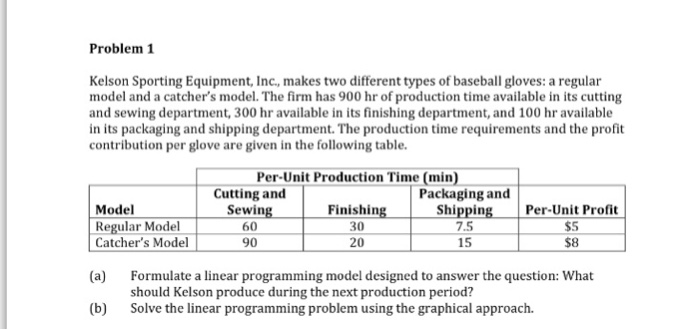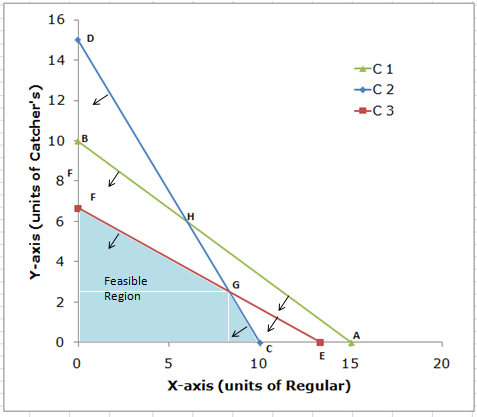# Question & Answer: Kelson Sporting Equipment, Inc., makes two different types of baseball gloves…..

Optimization problem.Kelson Sporting Equipment, Inc., makes two different types of baseball gloves: a regular model and a catcher’s model. The firm has 900 hr of production time available in its cutting and sewing department, 300 hr available in its finishing department, and 100 hr available in its packaging and shipping department. The production time requirements and the profit contribution per glove are given in the following table. (a) Formulate a linear programming model designed to answer the question: What should Kelson produce during the next production period? (b) Solve the linear programming problem using the graphical approach.

Decision Variables:

X = units of regular model of gloves to be produced

Don't use plagiarized sources. Get Your Custom Essay on
Question & Answer: Kelson Sporting Equipment, Inc., makes two different types of baseball gloves…..
GET AN ESSAY WRITTEN FOR YOU FROM AS LOW AS \$13/PAGE

Y = units of catcher’s model of gloves to be produced

Objective Function:

Objective is to maximize the total profit of producing both models of gloves. The profit function is given as follows:

Max Z = \$5X + \$8Y

Subject TO:

 Sr. No. Constraint Equation 1 Hours available with Cutting and Sewing department 60X + 90Y <= 900 2 Hours available with finishing department 30X + 20Y <= 300 3 Hours available with packaging and shipping department 7.5X + 15Y <= 100 4 Non-negative variables X, Y>= 0

Part B:

Graphical Solution:

Plotting of constraints on graph:

Convert the inequalities in the equality equation and find the X-axis and Y-axis intercepts to plot the equation lines.

 Sr. No. Constraints X-axis intercept (When Y = 0) Y-axis intercept (When X = 0) C1 60X + 90Y = 900 A (15, 0) B (0, 10) 30X + 20Y = 300 C (10, 0) D (0, 15) 7.5X + 15Y = 100 E (13, 0) F (0, 7)

The graph is shown below. The black arrows for constraint lines determine the feasible area for the respective constraints. The shaded region (blue) represents feasible region which satisfies all the constraints. The feasible region is OCGF.The point G is intersection of constraints C2 and C3, so solve the equation of both constraints by simultaneous method.

(C2 x 15) – (C3 x 20)

15(30X + 20Y) – 20(7.5X + 15Y) = (15 x 300) – (20 x 100)

450X + 600Y – 150X – 600Y = 4500 – 2000

300X = 2500

X = 8.33

Substitute X = 8.33 in C2

(30)(8.33) + 20Y = 300

250 + 20Y = 300

Y = 2.5

Coordinate of point G are (9.33, 2.5)

Determining Optimal Solution by extreme point’s method:

Compute objective value at each of the vertices of the feasible region as follows:

 Extreme Points Objective function Value (Z = 5X + 8Y) O (0, 0) 5 x 0 + 8 x 0 = 0 C (10, 0) 5 x 10 + 8 x 0 = 50 G (8.33, 2.5) 5 x 8.33 + 8 x 2.5 = 61.65 F (0, 7) 5 x 0 + 8 x 7 = 56

The point G maximizes the profit, thus optimal solution is at point G.

Units of regular model gloves = 8.33

Units of catcher’s model gloves = 2.5

Total profit = \$61.65